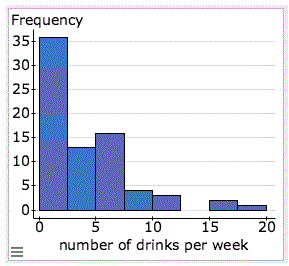# A group of 75 college students from a liberal arts college were randomly sampled and asked about the number of alcoholic drinks they have in a typical week. The file containing the data is linked below.

A group of 75 college students from a liberal arts college were randomly sampled and asked about the number of alcoholic drinks they have in a typical week. The file containing the data is linked below. The purpose of this study (Links to an external site.) was to compare the drinking habits of the students at the college to the drinking habits of college students in general. In particular, the dean of students, who initiated this study, would like to check whether the mean number of alcoholic drinks that students at his college have in a typical week differs from the mean of U.S. college students in general, which is estimated to be 4.73.

### Variables

The number of alcoholic beverages a student consumes in a week.

### Data: Hypothesis test results:

Variable Sample Mean Std. Err. DF T-Stat P-value
number of drinks per week 3.9333333 0.43592434 74 -1.8275343 0.0717

### Prompt

The drinks datafile is available in the Data section below.

1. Letμ be the mean number of alcoholic beverages that students in the liberal arts college drink in a typical week. State the hypotheses.
2. Here is a histogram of the data. Can we safely use the t-test with this data? Explain.3. Perform the t-test using StatCrunch. (directions)
Copy and paste the information from the StatCrunch output window into your initial post.
4. Based on the P-value, state your conclusions in context. Use a 5% level of significance.
NOTE: You do not need to double the P-value for this two-tailed test. StatCrunch calculates the P-value for you.
5. Using the context of this scenario, explain the meaning of each of following items from the StatCrunch output for the hypothesis test.
1. Std. Err (standard error)
2. T-stat (T-score)
3. P-value

1. The hypotheses are:

Ho:μ = 4.73

Ha:μ ≠ 4.73

2. Although the histogram is skewed to the right, we can still do a t-test because n=75 which is more than 30.

3.

### One sample T hypothesis test:

μ : Mean of variable
H0 : μ = 4.73
HA : μ ≠ 4.73

Hypothesis test results:

Variable Sample Mean Std. Err. DF T-Stat P-value
number of drinks per week 3.9333333 0.43592434 74 -1.8275343 0.0717

4. 2(0.0717)= 1.434

1.434>0.05

We do not have enough evidence to reject the null hypothesis that the average amount of alcoholic beverages that students at this college drink is the same as the national average.

5. a) Std. Err. is the standard error, which is an estimate of how far from the actual mean a sample mean is likely to be.

b) T-stat is T-score, which is used in a t-test to find evidence/lack of evidence of a significant difference between population means.

c) The P-value measures the likelihood of getting results as extreme as the sample in a population where the null hypothesis is true.